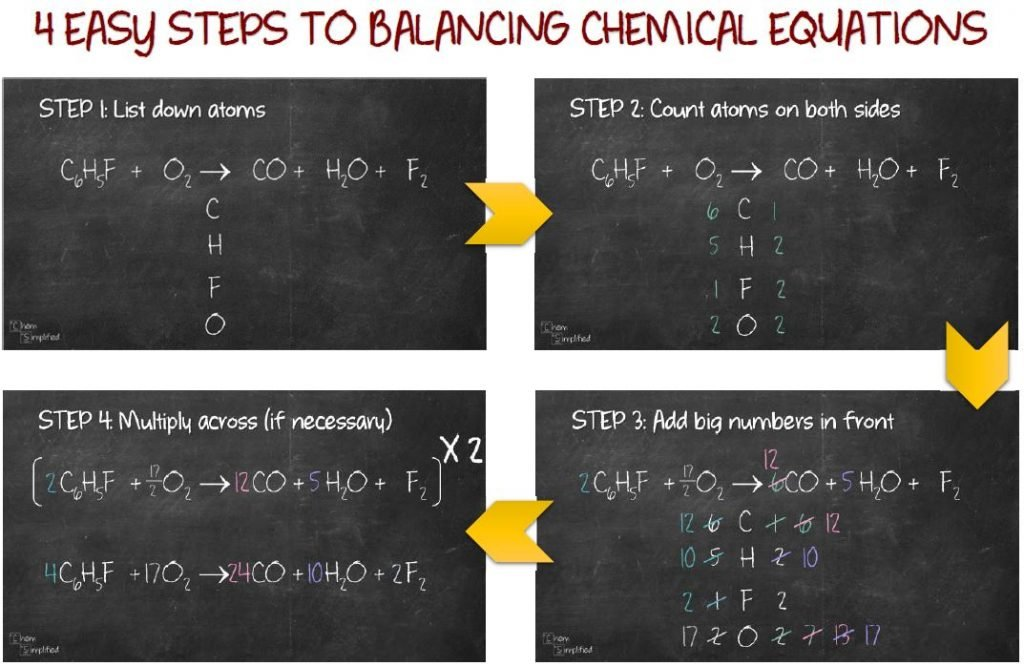# Balancing Chemical Equations

Is it important to be able to balance chemical equations correctly and quickly? You bet! Why so? First of all, you’ll make your chemistry teacher happy and maybe impress someone else along the way. Jokes aside, being able to balance chemical equation will open up a lot of possibilities. You’ll be able to get the molar ratios for all the compounds involved in the reaction and that will enable you to perform various types of calculations correctly (more on that in future posts).

So the main goal of balancing chemical equation is to get the number of atoms to be the same on each side of the equation. The compounds on the left-hand side (before the arrow) are called reactants while the compounds on the right-hand side (after the arrow) are called products.

NaCl + BeF2 → NaF + BeCl

If you count the number of atoms on both sides, you’ll notice that they are not equal:

• Na: 1 on left, 1 on right
• Cl: 1 on left, 2 on right
• Be: 1 on left, 1 on right
• F: 2 on left, 1 on right

There are 3 methods in mastering this art. Personally, I prefer Method 2 when it comes to solving easy to intermediate equations because it is simple and doesn’t tax my brain too much.

• Method 1: Inspection
• Method 2: Simple
• Method 3: Algebraic

Method 1: Inspection

Let’s go over the first method. It is exactly what the name states. You balance the equation by inspecting it. Let’s start with an example:

NaCl + BeF2 → NaF + BeCl

Na is balanced since there is one on the left-hand side and one on the right-hand side. So, we move on to Cl. Looks like it’s not balanced since there’s one on the left but two on the right. So, we’ll place a 2 in front of NaCl like this:

2 NaCl + BeF2 → NaF + BeCl

Since we added a 2 in front of NaCl, our Na on the right-hand side is no longer balanced, so we’ll need to add a 2 in front of NaF as well.

2 NaCl + BeF2 → 2 NaF + BeCl

Let’s do an atom count check.

• Na: 2 on left, 2 on right
• Cl: 2 on left, 2 on right
• Be: 1 on left, 1 on right
• F: 2 on left, 2 on right

Looks like the mission is accomplished!

This is a rather popular method and it works pretty well to a certain extent. However, it gets taxing when you encounter more complicated equation like this: C6H5F + O2 → CO + H2O + F2. You can take my word for it, or give it a try and see how long it’ll take you to balance this equation by inspection.

Method 2: Simple

We’ll try out the second method on this new equation. It pretty much works like the first method, however, you keep track of the changes by writing them down. There are 4 simple steps in balancing equation using this method. You’ll see how it’s done here:

Method 3: Algebraic

The final method is for those who loves solving algebraic equations or when you’re trying to balance complex equations (like the ones in Ninja level – see practice questions below). Basically, what you’ll do is set up a bunch of equations and then solve them to get the coefficients (numbers in front of the formula). I’ll walk you through the 4 steps involved in this video:

So, now that you’ve gone through the basics, how about testing out your skills? Each level below has 5 questions. Find out where you stand!

I would love to know which of the three methods you prefer. Vote below and see how it compares to others:

Coming SoonWhich method for balancing chemical equations do you prefer?
Which method for balancing chemical equations do you prefer?
Which method for balancing chemical equations do you prefer?

### 2 thoughts on “Balancing Chemical Equations”

1.